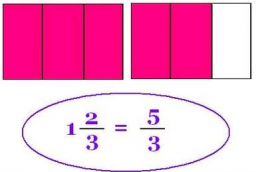# Comparing mixed numbers

Which of the following expression will give a sum of 7 and 3/10?
A. 3 and 1/5+ 4 and 2/2
B. 3 and 1/10+4 and 2/10
C. 1/10+ 7 and 2/5
D. 2 and 1/10+ 5 and 3/10

Result

x = (Correct answer is: B)### Step-by-step explanation:Did you find an error or inaccuracy? Feel free to write us. Thank you!

Tips to related online calculators
Need help to calculate sum, simplify or multiply fractions? Try our fraction calculator.